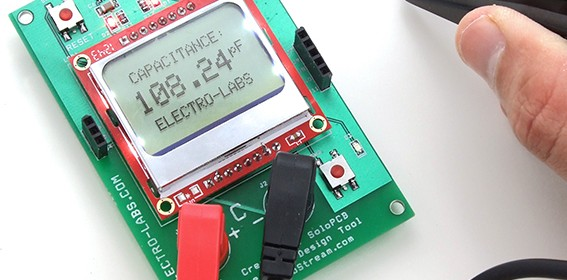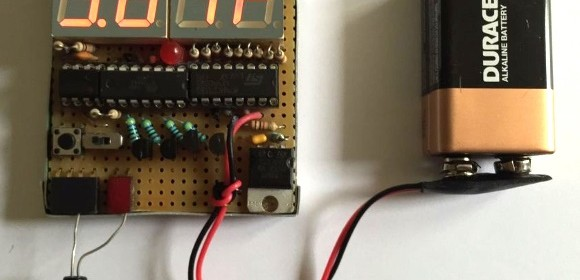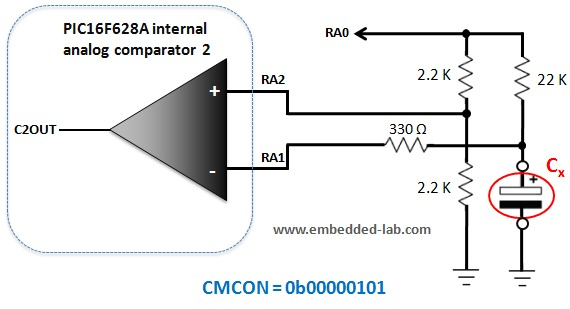## Arduino capacitance meterElectro-Labs’ DIY capacitance meter can measure capacitors rated from picofarads to millifarads. The circuit is based on Atmega328P and is Arduino IDE compatible. The capacitance is computed by measuring the capacitor charging time and is displayed on a Nokia 5110 LCD.

The main component of the circuit is Atmega328P microcontroller (MCU) and it runs at 16MHz. RESET, MOSI, MISO, SCK, RX, TX, 5V and GND pins are extended to the connectors from J4 to J11 to let the user use an Arduino or an AVR programmer to program the microcontroller in ICSP mode. MCU reset button, a general purpose button and a general purpose LED are also connected to the MCU. You can check out this tutorial to see how to burn a Arduino bootloader to a new microcontroller and then program it using the Arduino IDE.

There are two linear voltage regulators on the board. L78L05 and L78L05 generate 5V and 3.3V from 9V input. The MCU needs 5V and the LCD needs 3.3V to operate. The current consumption of the board is low, so no cooling is required.

## ATTINY84 based capacitance meterThomasVDD‘s DIY capacitance meter uses a 555 timer circuit as a monostable multivibrator, where the output pulse interval depends upon the value of the capacitance to me measured. An ATTiny84 is used to measure the duration of the 555 timer output pulse, and thus compute the capacitance, which is then displayed on a seven segment LED display module.

A while ago I also posted a tutorial on how to measure capacitance with a  PIC microcontroller.

## Making a digital capacitance meter using microcontrollerCapacitors are one of the most common passive electrical components that are extensively used in all kinds of electronic circuits. In this project, we will discuss a technique of building a digital capacitance meter using a PIC microcontroller. This project can measure capacitance values from 1 nF to 99 ?F, with a resolution of 1 nF. The technique is based on measuring the time elapsed when a capacitor is charged to a known voltage through a series resistor. The microcontroller used in this project is PIC16F628A.